## How to Calculate and Solve for Gas Pore Volume of a Reservoir Fluid Flow | The Calculator Encyclopedia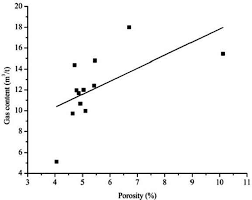The image above represents the gas pore volume.

To compute for the gas pore volume, three essential parameters are needed and these parameters are Initial Gas FVF (Bgi), Initial Water Saturation (Swi) and Initial Gas in Place (G).

The formula for calculating the gas pore volume:

(PV)g = GBgi / 1 – Swi

Where;

(PV)g = Gas Pore Volume
Bgi = Initial Gas FVF
Swi = Initial Water Saturation
G = Initial Gas in Place

Let’s solve an example;
Find the gas pore volume when the initial gas FVF is 12, initial water saturation is 9 and initial gas in place is 13.

This implies that;

Bgi = Initial Gas FVF = 12
Swi = Initial Water Saturation = 9
G = Initial Gas in Place = 13

(PV)g = GBgi / 1 – Swi
(PV)g = 13 x 12 / 1 – 9
(PV)g = 156 / -8
(PV)g =  -19.5

Therefore, the gas pore volume is -19.5

Calculating the Initial Gas FVF when the Gas Pore Volume, Initial Water Saturation and Initial Gas in Place is Given.

Bgi = (PV)g (1 – Swi) / G

Where;

Bgi = Initial Gas FVF
(PV)g = Gas Pore Volume
Swi = Initial Water Saturation
G = Initial Gas in Place

Let’s solve an example;
Find the initial gas FVF when the gas pore volume is 19, initial water saturation is 11 and initial gas in place is 16.

This implies that;

(PV)g = Gas Pore Volume = 19
Swi = Initial Water Saturation = 11
G = Initial Gas in Place = 16

Bgi = (PV)g (1 – Swi) / G
Bgi = 19 (1 – 11) / 16
Bgi = 19 (-10) / 16
Bgi = -190 / 16
Bgi = -11.875

Therefore, the initial gas FVF is -11.875.

Calculating the Initial Water Saturation when the Gas Pore Volume, Initial Gas FVF and Initial Gas in Place is Given.

Swi = 1 – GBgi / (PV)g

Where;

Swi = Initial Water Saturation
G = Initial Gas in Place
(PV)g = Gas Pore Volume
Bgi = Initial Gas FVF

Let’s solve an example;
Find the initial water saturation when the initial gas FVF is 24, initial gas in place is 6 and gas pore volume is 46.

This implies that;

G = Initial Gas in Place = 6
(PV)g = Gas Pore Volume = 46
Bgi = Initial Gas FVF = 24

Swi = 1 – GBgi / (PV)g
Swi = 1 – 6 x 24 / 46
Swi = 1 – 144 / 46
Swi = 1 – 3.13
Swi = -2.13

Therefore, the initial water saturation is -2.13.

## How to Calculate and Solve for Total Formation Volume Factor | The Calculator Encyclopedia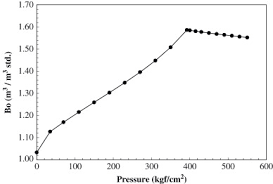The image above represents the total formation volume factor (FVF).

To compute for the total FVF, four essential parameters are needed and these parameters are oil FVF (Bo), gas FVF (Bg), initial gas solubility (Rsi) and current gas solubility (Rs).

The formula for calculating the total FVF:

BT = Bo + (Rsi – Rs)Bg

Where;

BT = Total FVF
Bo = Oil FVF
Bg = Gas FVF
Rsi = Initial Gas Solubility
Rs = Current Gas Solubility

Let’s solve an example;
Find the total FVF when the oil FVF is 14, gas FVF is 15, initial gas solubility is 26 and current gas solubility is 17.

This implies that;

Bo = Oil FVF = 14
Bg = Gas FVF = 15
Rsi = Initial Gas Solubility = 26
Rs = Current Gas Solubility = 17

BT = Bo + (Rsi – Rs)Bg
BT = 14 + (26 – 17)15
BT = 14 + (9)15
BT = 14 + 135
BT = 149

Therefore, the total FVF is 149.

Calculating the Oil FVF when the Total FVF, Gas FVF, Initial Gas Solubility and Current Gas Solubility is Given.

Bo = BT – (Rsi – Rs)Bg

Where;

Bo = Oil FVF
BT = Total FVF
Bg = Gas FVF
Rsi = Initial Gas Solubility
Rs = Current Gas Solubility

Let’s solve an example;
Find the oil FVF when the total FVF is 44, gas FVF is 17, initial gas solubility is 21 and current gas solubility is 19.

This implies that;

BT = Total FVF = 44
Bg = Gas FVF = 17
Rsi = Initial Gas Solubility = 21
Rs = Current Gas Solubility = 19

Bo = BT – (Rsi – Rs)Bg
Bo = 44 – (21 – 19)17
Bo = 44 – (2)17
Bo = 44 – 34
Bo = 10

Therefore, the oil FVF is 10.

Calculating the Gas FVF when the Total FVF, Oil FVF, Initial Gas Solubility and Current Gas Solubility is Given.

Bg = BT – Bo / Rsi – Rs

Where;

Bg = Gas FVF
Bo = Oil FVF
BT = Total FVF
Rsi = Initial Gas Solubility
Rs = Current Gas Solubility

Let’s solve an example;
Find the gas FVF when the total FVF is 48, oil FVF is 16, initial gas solubility is 20 and current gas solubility is 12.

This implies that;

Bo = Oil FVF = 16
BT = Total FVF = 48
Rsi = Initial Gas Solubility = 20
Rs = Current Gas Solubility = 12

Bg = BT – Bo / Rsi – Rs
Bg = 48 – 16 / 20 – 12
Bg = 32 / 8
Bg = 4

Therefore, the gas FVF is 4.

## How to Calculate and Solve for Total Oil Pore Volume of Reservoir Fluid Flow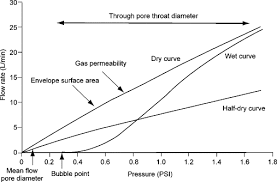The image above represents the total oil pore volume.

To compute for the total oil pore volume, four essential parameters are needed and these parameters are initial oil FVF (Boi), initial water saturation (Swi), oil in place (N) and gas cap ratio (m).

The formula for calculating the total oil pore volume:

(PV)o = NBoi(1 + m) / 1 + Swi

Where;

(PV)o = Total Oil Pore Volume
Boi = Initial Oil FVF
Swi = Initial Water Saturation
N = Oil in Place
m = Gas Cap Ratio

Let’s solve an example;
Find the total oil pore volume when the initial oil FVF is 9, the initial water saturation is 11, the oil in place is 21 and the gas cap ratio is 18.

This implies that;

Boi = Initial Oil FVF = 9
Swi = Initial Water Saturation = 11
N = Oil in Place = 21
m = Gas Cap Ratio = 18

(PV)o = NBoi(1 + m) / 1 + Swi
(PV)o = 21 x 9(1 + 18) / 1 + 11
(PV)o = 3591 / -10
(PV)o = -359.1

Therefore, the total oil pore volume is -359.1

## How to Calculate and Solve for Oil Saturation of Reservoir Fluid Flow | The Calculator Encyclopedia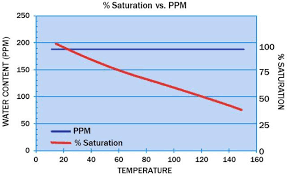The image above represents the oil saturation.

To compute for the oil saturation, five essential parameters are needed and these parameters are cumulative oil production (Np), oil in place (N), initial water saturation (Swi), initial oil FVF (Boi) and oil FVF (Bo).

The formula for calculating oil saturation:

S = [1 – Swi] [1 – Np / N] [Bo / Boi]

Where;

S = Oil Saturation
Np = Cumulative Oil Production
N = Oil in Place
Swi = Initial Water Saturation
Boi = Initial Oil FVF
Bo = Oil FVF

Let’s solve an example;
Given that the cumulative oil production is 22, oil in place is 8, initial water saturation is 16, initial oil FVF is 12 and the oil FVF is 7.
Find the oil saturation?

This implies that;

Np = Cumulative Oil Production = 22
N = Oil in Place = 8
Swi = Initial Water Saturation = 16
Boi = Initial Oil FVF = 12
Bo = Oil FVF = 7

S = [1 – Swi] [1 – Np / N] [Bo / Boi]
S = [1 – 16] [1 – 22/8] [7/12]
S = [1 – 16] [1 – 22/8] [0.583]
S = [1 – 16] [1 – 2.75] [0.583]
S = [1 – 16] [-1.75] [0.583]
S = [-15] [-1.75] [0.583]
S = 15.31

Therefore, the oil saturation is 15.31.

## How to Calculate and Solve for Cumulative GOR of Reservoir Fluid Flow | The Calculator Encyclopedia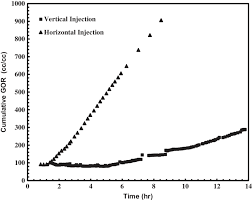The image above represents the cumulative GOR.

To compute for the cumulative GOR, two essential parameters are needed and these parameters are cumulative gas production (Gp) and cumulative oil production (Np).

The formula for calculating the cumulative GOR:

Rp = Gp / Np

Where;

Rp = Cumulative GOR
Gp = Cumulative Gas Production
Np = Cumulative Oil Production

Let’s solve an example;
Find the cumulative GOR with a cumulative gas production of 32 and a cumulative oil production of 44.

This implies that;

Gp = Cumulative Gas Production = 32
Np = Cumulative Oil Production = 44

Rp = Gp / Np
Rp = 32 / 44
Rp = 0.72

Therefore, the cumulative GOR is 0.72.

Calculating the Cumulative Gas Production when the Cumulative GOR and the Cumulative Oil Production is Given

Gp = Rp x Np

Where;

Gp = Cumulative Gas Production
Rp = Cumulative GOR
Np = Cumulative Oil Production

Let’s solve an example;
Find the cumulative gas production when the cumulative GOR is 56 and the cumulative oil production is 19.

This implies that;

Rp = Cumulative GOR = 56
Np = Cumulative Oil Production = 19

Gp = Rp x Np
Gp = 56 x 19
Gp = 1064

Therefore, the cumulative gas production is 1064.

## How to Calculate and Solve for Gas Recovery Factor (Production) | Reservoir Fluid Flow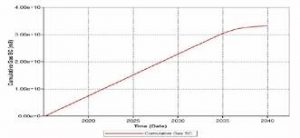The image above represents a gas recovery factor (G).

To compute for a gas recovery factor (production), two essential parameters are needed and these parameters are cumulative gas production (GP) and initial gas in place (G).

The formula for calculating the gas recovery factor (G):

RFG = Gp / G

Where:

RFG = Gas Recovery Factor (G)
GP = Cumulative Gas Production
G = Initial Gas in Place

Let’s solve an example;
Find the gas recovery factor (G) when the cumulative gas production is 13 and the initial gas in place is 17.

This implies that;

GP = Cumulative Gas Production = 13
G = Initial Gas in Place = 17

RFG = Gp / G
RFG = 13 / 17
RFG = 0.764

Therefore, the gas recovery factor (G) is 0.764.

Calculating the Cumulative Gas Production when the Gas Recovery Factor (G) and the Initial Gas in Place is Given.

GP = RFG x G

Where;

GP = Cumulative Gas Production
RFG = Gas Recovery Factor (G)
G = Initial Gas in Place

Let’s solve an example;
Find the cumulative gas production when the gas recovery factor (G) is 24 and the initial gas in place is 6.

This implies that;

RFG = Gas Recovery Factor (G) = 24
G = Initial Gas in Place = 6

GP = RFG x G
GP = 24 x 6
GP = 144

Therefore, the cumulative gas production is 144.

## How to Calculate and Solve for Gas Recovery Factor of a Reservoir Fluid Flow | The Calculator Encyclopedia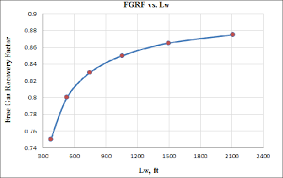The image above represents a gas recovery factor.

To compute for the gas recovery factor, three essential parameters are needed and these parameters are initial pressure(Pi), initial compressibility factor (Ziand P/Z Ratio (P/Z).

The formula for calculating the gas recovery factor:

RFG = 1 – P/Z.Pi / Zi

Where;

RFG = Gas Recovery Factor (P, Z)
Pi = Initial Pressure
Zi = Initial Compressibility Factor
P/Z = P/Z Ratio

Let’s solve an example;
Find the gas recovery factor when the initial pressure is 12, the initial compressibility factor is 7 and the P/Z ratio is 36.

This implies that;

Pi = Initial Pressure = 12
Zi = Initial Compressibility Factor = 7
P/Z = P/Z Ratio = 36

RFG = 1 – P / Z.Pi / Zi
RFG = 1 – 36[12 / 7]
RFG = 1 – 36[1.714]
RFG = 1 – 61.714
RFG = -60.714

Therefore, the gas recovery factor is -60.714.

## How to Calculate and Solve for Pressure and Initial Compressibility Factor Ratio of a Reservoir Fluid Flow | The Calculator Encyclopedia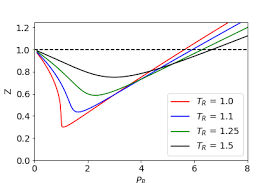The image above represents pressure and initial compressibility factor ratio (P/Z Ratio).

To compute for the pressure and initial compressibility factor ratio, four essential parameters are needed and these parameters are initial pressure (Pi), Initial Compressibility Factor (Zi), Cumulative Gas Production (GP) and initial gas in place (G).

The formula for calculating the P/Z Ratio:

P/Z = Pi / Zi (1 – Gp / G)

Where;

P/Z = P/Z Ratio
Pi = Initial Pressure
Zi = Initial Compressibility Factor
GP = Cumulative Gas Production
G = Initial Gas in Place

Let’s solve an example;
Given that the initial pressure is 20, the initial compressibility factor is 28, the cumulative gas production is 30 and the initial gas in place is 34.
Find the pressure and initial compressibility factor ratio (P/Z Ratio)?

This implies that;

Pi = Initial Pressure = 20
Zi = Initial Compressibility Factor = 28
GP = Cumulative Gas Production  = 30
G = Initial Gas in Place = 34

P/Z = Pi / Zi (1 – Gp / G)
P/Z = 20 / 28 (1 – 30 / 34)
P/Z = 20 / 28 (1 – 0.88)
P/Z = 20 / 28 (0.117)
P/Z = 0.714(0.117)
P/Z = 0.084

Therefore, the P/Z Ratio is 0.084.

Calculating for Initial Pressure when P/Z Ratio, Initial Compressibility Factor, Cumulative Gas Pressure and Initial Gas In Place is Given.

Pi = P/Z x Zi / (1 – Gp / G)

Where;

Pi = Initial Pressure
P/Z = P/Z Ratio
Zi = Initial Compressibility Factor
GP = Cumulative Gas Production
G = Initial Gas in Place

Let’s solve an example;
Given that the P/Z Ratio is 50, the initial compressibility factor is 18, the cumulative gas production is 25 and the initial gas in place is 32.
Find the initial pressure ?

This implies that;

P/Z = P/Z Ratio = 50
Zi = Initial Compressibility Factor = 18
GP = Cumulative Gas Production = 25
G = Initial Gas in Place = 32

Pi = P/Z x Zi / (1 – Gp / G)
Pi = 50 x 18 / (1 – 25 / 32)
Pi = 900 / (1 – 0.78125)
Pi = 900 / (0.21875)
Pi = 4114.28

Therefore, the Initial Pressure is 4114.28.

Calculating for Initial Compressibility Factor when P/Z Ratio, Initial Pressure, Cumulative Gas Pressure and Initial Gas In Place is Given.

Zi = Pi (1 – Gp / G) / P/Z

Where;

Zi = Initial Compressibility Factor
Pi = Initial Pressure
P/Z = P/Z Ratio
GP = Cumulative Gas Production
G = Initial Gas in Place

Let’s solve an example;
Given that the P/Z Ratio is 40, the initial pressure is 18, the cumulative gas production is 15 and the initial gas in place is 22.
Find the initial compressibility factor ?

This implies that;

Pi = Initial Pressure = 18
P/Z = P/Z Ratio = 40
GP = Cumulative Gas Production = 15
G = Initial Gas in Place = 22

Zi = Pi (1 – Gp / G) / P/Z
Zi = 18 (1 – 15 / 22) / 40
Zi = 18 (1 – 0.681) / 40
Zi = 18 (0.319) / 40
Zi = 5.742 / 40
Zi = 0.1435

Therefore, the initial compressibility factor is  0.1435.

## How to Calculate and Solve for Gas Initially in Place of a Reservoir Fluid Flow | The Calculator Encyclopedia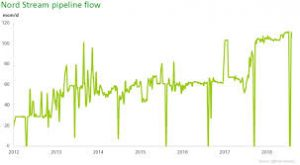The image above represents gas initially in place.

To compute for gas initially in place, five essential parameters are needed and these parameters are drainage area (A), initial gas FVF (Bgi), initial water saturation (Swi), porosity (φ) and thickness (h).

The formula for calculating gas initially in place:

G = 43560Ah φ (1 – Swi) / Bgi

Where;

G = Gas Initially in Place
A = Drainage Area
Bgi = Initial Gas FVF
Swi = Initial Water Saturation
φ = Porosity
h = Thickness

Let’s solve an example;
Find the gas initially in place with a drainage area of 44, initial gas FVF of 23, initial water saturation of 32, porosity of 18 and exponent of 14.

This implies that;

A = Drainage Area = 44
Bgi = Initial Gas FVF = 23
Swi = Initial Water Saturation = 32
φ = Porosity = 18
h = Thickness = 14

G = 43560Ah φ (1 – Swi) / Bgi
G = 43560 x 44 x 14 x 18 (1 – 32) / 23
G = 43560 x 44 x 14 x 18 (-31) / 23
G = -14972791680 / 23
G = -650990942.6

Therefore, the gas initially in place is -650990942.6

## How to Calculate and Solve for Gas Flow of Reservoir Fluid Flow | The Calculator Encyclopedia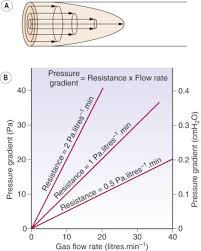The image above represents gas flow.

To compute for the gas flow, four essential parameters are needed and these parameters are Performance Co-efficient (C), Average Reservoir Pressure (Pr*), Flowing Bore Hole Pressure (Pwf*) and Exponent (n).

The formula for calculating the gas flow:

Qg = C(Pr*² – Pwf*²)n

Where;

Qg = Gas Flow
C = Performance Co-efficient
Pr* = Average Reservoir Pressure
Pwf* = Flowing Bore Hole Pressure
n = Exponent

Let’s solve an example;
Find the gas flow when a performance Co-efficient is 9, average reservoir pressure is 10, flowing bore hole pressure is 5 and an exponent of 20 is given.

This implies that;

C = Performance Co-efficient = 9
Pr* = Average Reservoir Pressure = 10
Pwf* = Flowing Bore Hole Pressure = 5
n = Exponent = 20

Qg = C(Pr*² – Pwf*²)n
Qg = 9(10² – 5²)20
Qg = 9(100 – 25)20
Qg = 9(75)20
Qg = 9(3.17e+37)
Qg = 2.85e+38

Therefore, the gas flow is 2.85e+38.